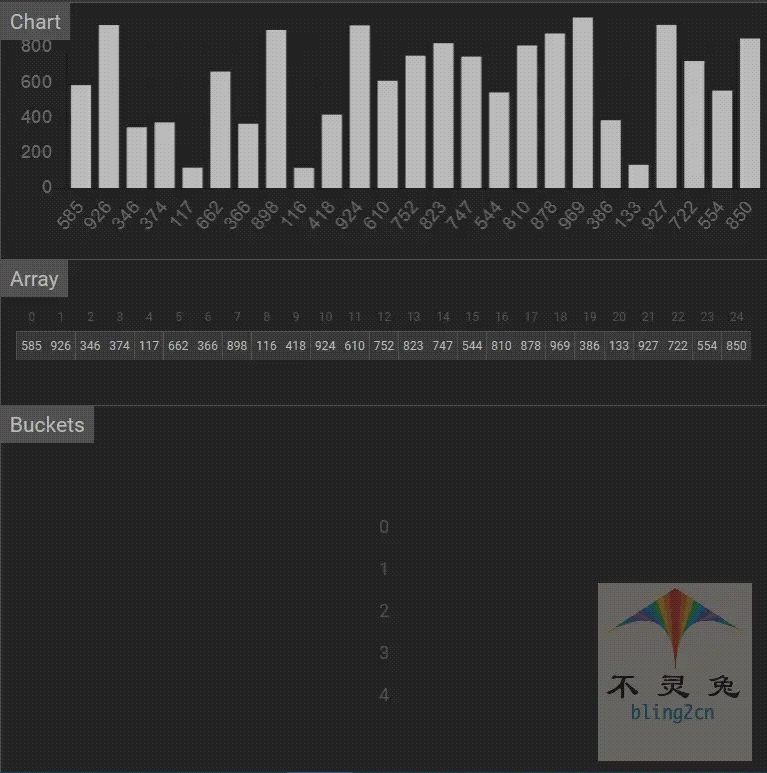# Python implementation ten bucket sorting algorithm (Bucket Sort)

Posted May 28, 20203 min read

## Introduction

Bucket Sort(Bucket Sort), also called box sorting, whose main idea is:store the elements in the same value range in the set to be sorted into the same bucket, that is, split the set into multiple according to the characteristics of the element value Areas, multiple buckets formed after splitting, are in order from the range of values. To sort the elements in each bucket, the set of elements in all buckets is sorted.

Bucket sorting is an extended version of counting sorting. Counting sorting can be seen as storing only the same elements in each bucket, while bucket sorting stores a certain range of elements in each bucket. Bucket sorting needs to ensure that the elements are dispersed evenly, otherwise, when all the data is concentrated in the same bucket, the bucket sorting fails.

## Algorithm implementation steps

1. Determine the number of buckets applied according to the difference range and mapping rules of the largest and smallest elements in the set to be sorted;
2. Traverse the sorting sequence and put each element in the corresponding bucket;
3. Sort buckets that are not empty;
4. Access the buckets in order, and put the elements in the buckets back to the corresponding positions in the original sequence in order to complete the sorting.

## Python code implementation

``````# bucket_sort code implementation

from typing import List

def bucket_sort(arr:List [int]):
"" "Bucket Sorting" ""
min_num = min(arr)
max_num = max(arr)
# Bucket size
bucket_range =(max_num-min_num)/len(arr)
# Bucket array
count_list = [[]for i in range(len(arr) + 1)]
# Fill the bucket array
for i in arr:
count_list [int((i-min_num) //bucket_range)]. append(i)
arr.clear()
# Backfill, the sort inside the bucket directly calls sorted
for i in count_list:
for j in sorted(i):
arr.append(j)

# Test Data

if __name__ == '__main__':
import random
random.seed(54)
arr = [random.randint(0,100) for _ in range(10)]
print("raw data:", arr)
bucket_sort(arr)
print("Bucket sorting result:", arr)

# Output result

Original data:[17, 56, 71, 38, 61, 62, 48, 28, 57, 42]
Bucket sorting results:[17, 28, 38, 42, 48, 56, 57, 61, 62, 71]``````

## Animated demo## Analysis of Algorithms

• time complexity

Best case:The input sequence is sorted, and the time complexity of insertion sort is \$O(n) \$, that is, the best time complexity is \$O(n) \$.

Worst case:For the sequence to be sorted, the size is \$n \$, which is divided into \$k \$buckets, and \$n \$cycles need to be performed, each element is loaded into the corresponding bucket; \$k \$cycles, for each Sort the data in each bucket(average \$n/k \$elements per bucket).

If a quick sort algorithm is used for sorting, the average time complexity of a single sort is \$O(nlog \ _2n) \$, and the worst time complexity is \$O(n ^ 2) \$. The bucket sorting process is inserted in the form of a linked list, so the time complexity of the entire bucket sorting is:

\$\$Average time complexity:O \ left(n \ right) + O \ left(k \ times \ left(\ frac {n} {k} \ log \ _2 \ left(\ \ frac {n} {k} \ right) \ right) \ right) = O \ left(n \ times \ left(\ log \ _2 \ left(\ frac {n} { k} \ right) +1 \ right) \ right) \\ Worst time complexity:O \ left(n \ right) + O \ left(k \ times \ left(\ frac {n} {k} \ right) ^ 2 \ right) = O \ left(n + \ frac {n ^ 2} {k} \ right) \$\$

When \$n = k \$, the time complexity is at least \$O(n) \$; when \$k = 1 \$, the time complexity is at most \$O(n + n ^ 2) \$.

So the worst time complexity is:\$O(n ^ 2) \$.

Average case:The average time complexity is \$O(n) \$.

• Space complexity

In the bucket sorting process, additional space of \$k \$buckets and extra space of \$n \$elements are created, so the space complexity of bucket sorting is \$O(n + k) \$.

• Stability

The stability of bucket sorting depends on the algorithm used for sorting in the bucket. If it is a queue, the relative position of the same element extraction and replacement can be ensured, that is, the sorting is stable, and if the stack is used, the sorting must be unstable. Since bucket sorting can be stabilized, bucket sorting is a stable sorting algorithm.

• Overview

Time complexity(average) Time complexity(best) Time complexity(worst) Space complexity Sort by Stability
\$O(n) \$ \$O(n) \$ \$O(n ^ 2) \$ \$O(n + k) \$ out-place stable